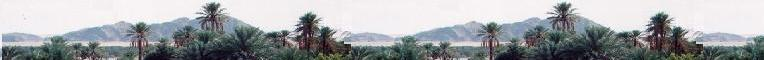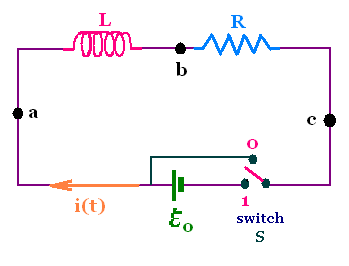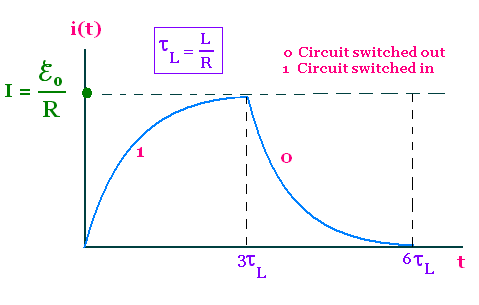Electrostatics

Electromagnetics

Electricity &
Magnetism

# Self-inductance and LR circuit

### 1. Self-induced emf's

Consider a loop or coil carrying a current i. Then it exists, in the vicinity of the loop, an induced magnetic field B given by the Biot-Savart law. The induced field is proportional to the current i. The changing magnetic flux Φ linking the loop produces the emf given by Faraday's law ℰ = - dΦB/dt. We obtain a varying flux by moving a magnet. But the varying magnetic flux can be also obtained by varying B then by varying the current i. This process of changing the current in a circuit (loop's or coil's own current) produces a self-induced emf.

If the magnetic field B induced is proportional to i, then does the magnetic flux. We write Φ = L i

L = - dΦB/dt = - L di/dt

The constant of proportionality L is defined as the self-inductance (or inductance) for the loop.

The SI unit of inductance in the Henry (H). It is a large unit of inductance. the values of self-inductance of coils in electronic circuits are typically in the 1µH to a mH range.

### 2. Example of a solenoid

Solenoid of length l, and cross-sectional area S with n turns per unit length. The magnitude of the field is B = μo n i. The flux linking one loop is ΦB = μo n i S. The flux linking a solenoid is NΦB , where N = nl.
The emf induced in the N-turn coil solenoid is ℰL = - N dΦB/dt = - nl μo n S di/dt

Therefore

L = μon2Sl

L = μon2Sl

That is the self-inductance of an ideal (infinitely long) solenoid, where we neglect the end effects.

The inductance can be measured in a circuit that contain the related element as a coil.

### 3. L R circuit

##### 3.1. Switch at the position 1

At the position 1, the battery is connected; the current increases during a certain time until it stabilizes and becomes equal to the steady value I = ℰo/R.Consider a circuit with a coil or solenoid providing a self-inductance connected to a battery through a switch. When the switch is closed, the battery causes charge to move in the circuit. The solenoid has a self-inductance L and a resistance R.

As the current i(t) in the circuit changes through the switch, there is a self-induced emf ℰL = - L di(t)/dt in the inductance that opposes a change in the current, preventing it to vary abruptly. The instantaneous value of the current depends on the value of L, R and the emf of the battery ℰo.

We apply the loop rule: Starting at the point a, proceeding clockwise, and equating the sum of the voltage change to zero gives:

(Va - Vc) + (Vb - Va) + (Vc - Vb) = 0

We have:

Va - Vc = ℰo
Vb - Va = - L di/dt
Vc - Vb = - R i

Therefore:

o - L di/dt - Ri = 0
- L di/dt = Ri - ℰo = R(i - ℰo/R)
We obtain the following differential equation:
di/(i - ℰo/R) = - (R/L )dt
Integrating, we obtain:
i(t) = Const. exp { - (R/L)t} + ℰo/R
Where Const is a constant integration.

We find this constant Const by applying the initial condition i(0) = 0:
At t = 0, i(0) = 0 = Const + ℰo/R

Thus

Const = - ℰo/R

We define a characteristic time parameter: τL = L/R called the inductive time constant.
i(t) = - (ℰo/R) exp {- t/τL} + ℰo/R

i(t) = (ℰo/R) (1 - exp {- t/τL})

Instantaneous increasing current through
an inductance: τL = L/R

i(t) = (ℰo/R) (1 - exp {- t/τL})

At a long time (t → ∞) i(t) becomes equal to the steady value I = ℰo/R, that is the value of the current i without the inductance. The inductive time constant τL = L/R sets the time scale for the LR circuit. At t = 5τL, the value of the current i(t) is already 0.99 ℰo/R.

##### 3.1. Switch at the position 0

At the position 0, the battery is disconnected; the current decreases during a certain time until it vanishes, and becomes equal to the steady value I = 0.

At the time t = 0, while the current has the value io, we disconnect the battery. Thus the loop rule, with ℰo is :

0 - L di/dt - Ri = 0.

Solving this differential equation, using the initial condition i(0) = io, gives:

i(t) = ioexp {- t/τL}

Instantaneous decreasing current through
an inductance: τL = L/R

i(t) = ioexp {- t/τL}

At the time t = τL, the current has dropped to ioexp {- 1} = 0.37io. At t = 3τL, the current becomes practically zero.### 4. Energy transfers in LR circuits

##### 4.1. Energy stored in an inductor

The gravitational potential energy of a mass changes as the mass moves through a gravitational field.

The electric potential energy of charge carriers changes as carriers move through a potential difference in a circuit element.

Consider an inductor, of ohmic resistance r and inductance L. In addition to the Joule heating in the inductor at the rate Pr = ri2, there is also an electric potential energy UL due the self-induced emf ℰ.

If the current is increasing in the inductor, the self-induced emf opposing the current is negative, hence the carriers lose potential energy.

If the current is decreasing in the inductor, the self-induced emf opposing the current is positive, hence the carriers gain potential energy.

The potential energy lost by the charge carriers when the current is increasing is maintained within or stored in the inductor due to the change in the potential difference between the conductor.

The potential energy gained by the charge carriers when the current is decreasing is returned or restored or recovered by the inductor due to the change in the potential difference between the conductor.

Each time the potential energy if transformed to and from a stored energy in the inductor, called the magnetic energy of the inductor.

The power P, or rate of energy used or transformed in the inductor is P = dUL/dt, where dUL is the infinitesimal electric potential energy change stored in the inductor. This change dU corresponds to the change di in current.

We have P = dUL/dt = ℰi = i L (di/dt)

P = Li(di/dt)

Since dUL/dt = i L (di/dt), or

dUL = L i di, we integrate to obtain

UL(t) = (1/2) L i2(t) + const.

At t = 0, UL(t) = 0. Hence const = 0.

Therefore the energy stored in the inductor is:

UL = (1/2) L i2

Energy stored in an inductor:
UL = (1/2) L i2

##### 4.1. Energy stored in an inductor

Consider a solenoid of length l, and cross-sectional area S with n turns per unit length. Its inductance is: L = μon2Sl. When crossed by a current I, the produced magnetic field is B = μonI.

Therefore

UL = (1/2) L i2 = (1/2) μon2Sl i2 =
(1/2) μon2Sl (B/μon)2 =
(1/2) Sl B2 (1/μo) = Sl B2/2μo

The product Sl represents the volume of the space inside the solenoid. Hence the energy density stored in the solenoid is uB = UL/V = B2/2μo

Energy density stored in the magnetic field of an ideal solenoid:
uB = B2/2μo

This result valid for a solenoid is in fact general. The energy density of an inductor is equal to the half of the square of its induced magnetic field divided by the vacuum permeability.

 chimie labs | Physics and Measurements | Probability & Statistics | Combinatorics - Probability | Chimie | Optics | contact |